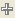To suppress formula evaluation, click the red triangle menu next to the keypad and select Suppress Eval. When evaluation is suppressed, the formula iconappears dimmed.
 • If the icon appears to the right of the red triangle menu in the Formula Editor, it indicates that formula evaluation is suppressed for that formula.
 • You can turn off formula evaluation for all formulas by selecting Suppress Formula Eval from the data table red triangle menu. To re-evaluate formulas, select Rerun Formulas from the data table red triangle menu. You can also click the Apply button in the Formula Editor for each column that contains a pending formula.
 • When you turn off formula evaluation, edit a formula, and click Apply, you are prompted for whether to evaluate the formula. To allow formula evaluation once, click Evaluate formula this time.

Help created on 7/12/2018